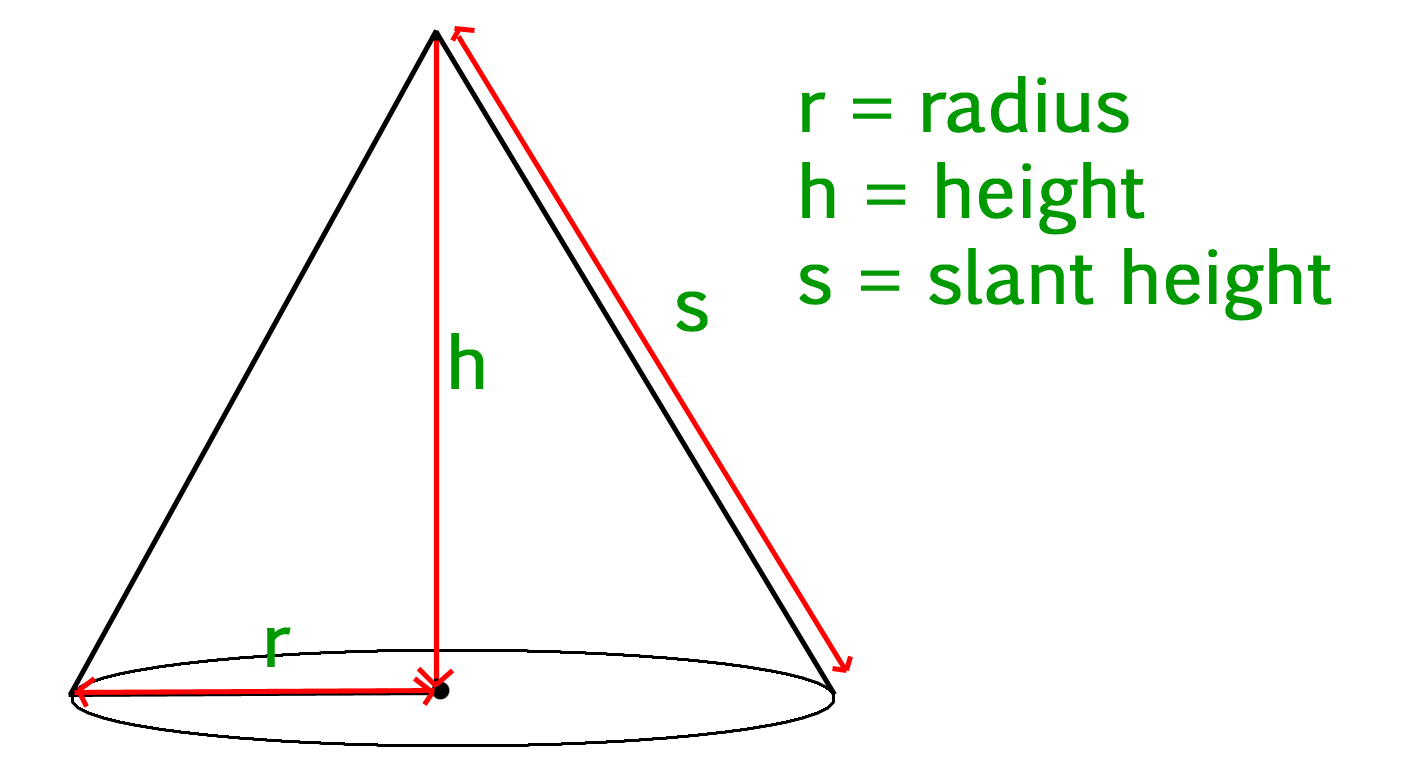GeeksforGeeks App
Open AppBrowser
Continue

# Calculate volume and surface area of a cone

Given slant height, height and radius of a cone, we have to calculate the volume and surface area of the cone.

• Cone :
Cone is a three dimensional geometric shape. It consists of a base having the shape of a circle and a curved side (the lateral surface) ending up in a tip called the apex or vertex.• Volume of a cone :
The volume of a cone is given by the formula –

`volume = 1/3(pi * r * r * h)`
• where r is the radius of the circular base, and h is the height (the perpendicular distance from the base to the vertex).

• Surface area of a cone :
The surface area of a cone is given by the formula –

`area = pi * r * s + pi * r^2  `
• Where r is the radius of the circular base, and s is the slant height of the cone.

Examples :

```Input :
slant_height = 13
height = 12
Output :
Volume Of Cone = 314.159
Surface Area Of Cone = 282.743

Input :
slant_height = 10
height = 8
Output :
Volume Of Cone = 301.593
Surface Area Of Cone = 301.593```

## C++

 `// CPP program to calculate Volume ``// and Surface area of Cone``#include``using` `namespace` `std;`` ` `float` `pi = 3.14159;`` ` `// Function to calculate``// Volume of cone``float` `volume(``float` `r, ``float` `h)``{``    ``return` `(``float``(1) / ``float``(3)) * pi * ``                             ``r * r * h;``}`` ` `// Function to calculate``// Surface area of cone``float` `surface_area(``float` `r, ``float` `s)``{``    ``return` `pi * r * s + pi * r * r;``}`` ` `// Driver Code``int` `main()``{``    ``float` `radius = 5;``    ``float` `slant_height = 13;``    ``float` `height = 12;``    ``float` `vol, sur_area;`` ` `    ``// Printing value of volume ``    ``// and surface area``    ``cout << ``"Volume Of Cone : "``        ``<< volume(radius, height) << endl;``    ``cout << ``"Surface Area Of Cone : "``        ``<< surface_area(radius, slant_height);``    ``return` `0;``}`

## Java

 `// Java program to calculate ``// Volume and Surface area of cone``class` `GFG ``{``    ``static` `float` `pi = ``3``.14159f;`` ` `    ``// Function to calculate ``    ``// Volume of cone``    ``public` `static` `float` `volume(``float` `r, ``                               ``float` `h)``    ``{``        ``return` `(``float``)``1` `/ ``3` `* pi * h *``                               ``r * r;``    ``}`` ` `    ``// Function to calculate ``    ``// Surface area of cone``    ``public` `static` `float` `surface_area(``float` `r, ``                                     ``float` `s)``    ``{``        ``return` `pi * r * s + pi * r * r;``    ``}`` ` `    ``// Driver Code``    ``public` `static` `void` `main(String args[])``    ``{``        ``float` `radius = ``5``;``        ``float` `slant_height = ``13``;``        ``float` `height = ``12``;``        ``float` `vol, sur_area;`` ` `        ``// Printing value of volume``        ``// and surface area``        ``System.out.print(``"Volume Of Cone : "``);``        ``System.out.println(volume(radius, height));`` ` `        ``System.out.print(``"Surface Area Of Cone : "``);``        ``System.out.println(surface_area(radius, ``                                        ``slant_height));``         ` `    ``}``}`` ` `// This code is contributed by "akanshgupta"`

## Python

 `''' Python3 program to calculate Volume and ``    ``Surface area of Cone'''`` ` `# Importing Math library for value Of PI``import` `math``pi ``=` `math.pi`` ` `# Function to calculate Volume of Cone``def` `volume(r, h):``    ``return` `(``1` `/` `3``) ``*` `pi ``*` `r ``*` `r ``*` `h`` ` `# Function To Calculate Surface Area of Cone``def` `surfacearea(r, s):``    ``return` `pi ``*` `r ``*` `s ``+` `pi ``*` `r ``*` `r`` ` `# Driver Code``radius ``=` `float``(``5``)``height ``=` `float``(``12``)``slat_height ``=` `float``(``13``)``print``( ``"Volume Of Cone : "``, volume(radius, height) )``print``( ``"Surface Area Of Cone : "``, surfacearea(radius, slat_height) )`

## C#

 `// C# program to calculate ``// Volume and Surface area of cone``using` `System;`` ` `class` `GFG ``{``    ``static` `float` `pi = 3.14159f;`` ` `    ``// Function to calculate ``    ``// Volume of cone``    ``public` `static` `float` `volume(``float` `r, ``                               ``float` `h)``    ``{``        ``return` `(``float``)1 / 3 * pi * h * ``                               ``r * r;``    ``}`` ` `    ``// Function to calculate ``    ``// Surface area of cone``    ``public` `static` `float` `surface_area(``float` `r, ``                                     ``float` `s)``    ``{``        ``return` `pi * r * s + pi * r * r;``    ``}`` ` `    ``// Driver Code``    ``public` `static` `void` `Main()``    ``{``        ``float` `radius = 5;``        ``float` `slant_height = 13;``        ``float` `height = 12;``        ``//float vol, sur_area;`` ` `        ``// Printing value of volume ``        ``// and surface area``        ``Console.Write(``"Volume Of Cone : "``);``        ``Console.WriteLine(volume(radius, ``                                 ``height));`` ` `        ``Console.Write(``"Surface Area Of Cone : "``);``        ``Console.WriteLine(surface_area(radius, ``                                       ``slant_height));``         ` `    ``}``}`` ` `// This code is contributed by "vt_m"`

## PHP

 ``

## Javascript

 ``

Output :

```Volume Of Cone : 314.159
Surface Area Of Cone : 282.743```

Time complexity : O(1)
Auxiliary Space : O(1)

My Personal Notes arrow_drop_up